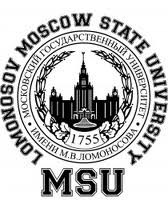Publications

Numerical modelling of bio-membranes

In this recently launched project we approach modeling of bio-membranes basing on the laws of continuum mechanics and thermodynamics . We further use numerical simulations to visualize, understand and predict.

Collaborators:
Sheereen Majd (University of Houston)
Annalisa Quaini (University of Houston)

Lateral phase separation

This example is motivated by the computational study of lipid bilayer phase behavior. In the continuum model for phase separation in a lipid bilayer the two-component membrane is modeled by a closed, sufficiently smooth surface $\Gamma\subset\mathbb{R}^3$, on which two species with mass concentrations $\psi_1, \psi_2 \in L^2(\Gamma)$ form a heterogeneous mixture. The conservation of the representative concentration $\psi$ (e.g., $\psi = \psi_1$) on $\Gamma$ together with minimization of the total specific free energy functional $\int_\Gamma f(\psi)ds$, with $f(\psi) = f_0(\psi) + \frac{1}{2} \epsilon^2 | \nabla_\Gamma \psi |^2$, leads to the surface Cahn--Hilliard equation: \begin{align}\label{eq:CH} \rho \psi_t - \mbox{div}_\Gamma \left(M \nabla_\Gamma \left(f_0' - \epsilon^2 \Delta_\Gamma \psi\right)\right) = 0 \quad \text{on}~\Gamma, \end{align} where $\rho$ is the density, $M$ is the mobility coefficient, $f_0(\psi)$ is the free energy per unit surface, e.g. in the form of double-well potential $f_0(\psi)=\frac14\psi^2(1-\psi)^2$, and ${\epsilon}$ is the size of the interface between the two phases.

Examples of spinodal decomposition and lateral separation for conserved phases on biologically relevant steady shapes from . All simulation were done with TraceFEM.

Spinodal decomposition and phase separation on the sphere (left), on the spindle (center), on amorphous shape (right). Simulated using conservative Cahn-Hilliard model.

The minimization of the total specific free energy functional with phase transition (no conservation) leads to the surface Allen--Cahn equation: \begin{align} \psi_t + M (f_0' - \epsilon^2 \Delta_\Gamma \psi) = 0 \quad \text{on}~\Gamma, \end{align}
Examples of spinodal decomposition and lateral separation with phases transition on biologically relevant steady shapes from . All simulation were done with TraceFEM.

Spinodal decomposition and phase separation on the sphere (left), on the spindle (center), on amorphous shape (right). Simulated using non-conservative Allen-Cahn model.

Two phases are in the thermodynamic equilibrium:
• on each of two spheres until they start merging (left),
• on the sphere surface until it starts splitting into two (right).
The animation visualizes the solution to the surface Cahn-Hilliard equations (see  for details).

The bibliography

(this is by no means complete list of papers on the topic; it collects only papers I am co-authoring)

1. V.  Yushutin, A. Quaini, S. Majd, M. Olshanskii, A computational study of lateral phase separation in biological membranes, International Journal for Numerical Methods in Biomedical Engineering, V. 35 (2019), e3182;  doi:10.1002/cnm.3181pdf-file;

2. V.  Yushutin, A. Quaini, M. Olshanskii, Numerical modelling of phase separation on dynamic surfaces, Journal of Computational Physics; V.  407 (2020), Article 109126;   doi:10.1016/j.jcp.2019.109126, pdf-file;

**Current support acknowledgment: NSF through the Division of Mathematical Sciences grant 1717516.

 Address: Department of Mathematics University of Houston 651 PGH Houston, Texas 77204 tel:+1-713-743 3500 email: molshan (at) math.uh.edu